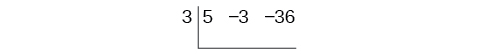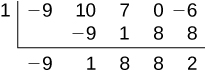# 5.4 Dividing polynomials  (Page 3/6)

 Page 3 / 6

## Using synthetic division to divide a second-degree polynomial

Use synthetic division to divide $\text{\hspace{0.17em}}5{x}^{2}-3x-36\text{\hspace{0.17em}}$ by $\text{\hspace{0.17em}}x-3.\text{\hspace{0.17em}}$

Begin by setting up the synthetic division. Write $\text{\hspace{0.17em}}k\text{\hspace{0.17em}}$ and the coefficients.Bring down the lead coefficient. Multiply the lead coefficient by $\text{\hspace{0.17em}}k.\text{\hspace{0.17em}}$Continue by adding the numbers in the second column. Multiply the resulting number by $\text{\hspace{0.17em}}k.\text{\hspace{0.17em}}$ Write the result in the next column. Then add the numbers in the third column.The result is $\text{\hspace{0.17em}}5x+12.\text{\hspace{0.17em}}$ The remainder is 0. So $\text{\hspace{0.17em}}x-3\text{\hspace{0.17em}}$ is a factor of the original polynomial.

## Using synthetic division to divide a third-degree polynomial

Use synthetic division to divide $\text{\hspace{0.17em}}4{x}^{3}+10{x}^{2}-6x-20\text{\hspace{0.17em}}$ by $\text{\hspace{0.17em}}x+2.\text{\hspace{0.17em}}$

The binomial divisor is $\text{\hspace{0.17em}}x+2\text{\hspace{0.17em}}$ so $\text{\hspace{0.17em}}k=-2.\text{\hspace{0.17em}}$ Add each column, multiply the result by –2, and repeat until the last column is reached.The result is $\text{\hspace{0.17em}}4{x}^{2}+2x-10.\text{\hspace{0.17em}}$ The remainder is 0. Thus, $\text{\hspace{0.17em}}x+2\text{\hspace{0.17em}}$ is a factor of $\text{\hspace{0.17em}}4{x}^{3}+10{x}^{2}-6x-20.\text{\hspace{0.17em}}$

## Using synthetic division to divide a fourth-degree polynomial

Use synthetic division to divide $\text{\hspace{0.17em}}-9{x}^{4}+10{x}^{3}+7{x}^{2}-6\text{\hspace{0.17em}}$ by $\text{\hspace{0.17em}}x-1.\text{\hspace{0.17em}}$

Notice there is no x -term. We will use a zero as the coefficient for that term.The result is $\text{\hspace{0.17em}}-9{x}^{3}+{x}^{2}+8x+8+\frac{2}{x-1}.$

Use synthetic division to divide $\text{\hspace{0.17em}}3{x}^{4}+18{x}^{3}-3x+40\text{\hspace{0.17em}}$ by $\text{\hspace{0.17em}}x+7.$

$3{x}^{3}-3{x}^{2}+21x-150+\frac{1,090}{x+7}$

## Using polynomial division to solve application problems

Polynomial division can be used to solve a variety of application problems involving expressions for area and volume. We looked at an application at the beginning of this section. Now we will solve that problem in the following example.

## Using polynomial division in an application problem

The volume of a rectangular solid is given by the polynomial $\text{\hspace{0.17em}}3{x}^{4}-3{x}^{3}-33{x}^{2}+54x.\text{\hspace{0.17em}}$ The length of the solid is given by $\text{\hspace{0.17em}}3x\text{\hspace{0.17em}}$ and the width is given by $\text{\hspace{0.17em}}x-2.\text{\hspace{0.17em}}$ Find the height, $\text{\hspace{0.17em}}t,$ of the solid.

There are a few ways to approach this problem. We need to divide the expression for the volume of the solid by the expressions for the length and width. Let us create a sketch as in [link] .

We can now write an equation by substituting the known values into the formula for the volume of a rectangular solid.

$\begin{array}{ccc}\hfill V& =& l\cdot w\cdot h\hfill \\ \hfill 3{x}^{4}-3{x}^{3}-33{x}^{2}+54x& =& 3x\cdot \left(x-2\right)\cdot h\hfill \end{array}$

To solve for $\text{\hspace{0.17em}}h,\text{\hspace{0.17em}}$ first divide both sides by $\text{\hspace{0.17em}}3x.$

$\begin{array}{ccc}\hfill \frac{3x\cdot \left(x-2\right)\cdot h}{3x}& =& \frac{3{x}^{4}-3{x}^{3}-33{x}^{2}+54x}{3x}\hfill \\ \hfill \left(x-2\right)h& =& {x}^{3}-{x}^{2}-11x+18\hfill \end{array}$

Now solve for $\text{\hspace{0.17em}}h\text{\hspace{0.17em}}$ using synthetic division.

$h=\frac{{x}^{3}-{x}^{2}-11x+18}{x-2}$The quotient is $\text{\hspace{0.17em}}{x}^{2}+x-9\text{\hspace{0.17em}}$ and the remainder is 0. The height of the solid is $\text{\hspace{0.17em}}{x}^{2}+x-9.$

The area of a rectangle is given by $\text{\hspace{0.17em}}3{x}^{3}+14{x}^{2}-23x+6.\text{\hspace{0.17em}}$ The width of the rectangle is given by $\text{\hspace{0.17em}}x+6.\text{\hspace{0.17em}}$ Find an expression for the length of the rectangle.

$3{x}^{2}-4x+1$

Access these online resources for additional instruction and practice with polynomial division.

## Key equations

 Division Algorithm

## Key concepts

• Polynomial long division can be used to divide a polynomial by any polynomial with equal or lower degree. See [link] and [link] .
• The Division Algorithm tells us that a polynomial dividend can be written as the product of the divisor and the quotient added to the remainder.
• Synthetic division is a shortcut that can be used to divide a polynomial by a binomial in the form $\text{\hspace{0.17em}}x-k.\text{\hspace{0.17em}}$ See [link] , [link] , and [link] .
• Polynomial division can be used to solve application problems, including area and volume. See [link] .

More example of algebra and trigo
What is Indices
If one side only of a triangle is given is it possible to solve for the unkown two sides?
please I need help in maths
Okey tell me, what's your problem is?
Navin
the least possible degree ?
(1+cosA)(1-cosA)=sin^2A
good
Neha
why I'm sending you solved question
Mirza
Teach me abt the echelon method
Khamis
exact value of cos(π/3-π/4)
What is differentiation?
modul questions trigonometry
(1+cosA)(1-cosA)=sin^2A
BINCY
differentiate f(t)=1/4t to the power 4 +8
I need trigonometry,polynomial
ok
Augustine
Why is 7 on top
simplify cot x / csc x
👉🌹Solve🌻 Given that: cotx/cosx =cosx/sinx/cosx =1/sinx =cosecx Ans.
Vijay
what is the period of cos?
Patrick
simplify: cot x/csc x
Catherine
sorry i didnt realize you were actually asking someone else to put their question on here. i thought this was where i was supposed to.
Catherine
some to dereve formula for bulky density
kurash
Solve Given that: cotx/cosx =cosx/sinx/cosx =1/sinx =cosecx Ans.
Vijay
if tan alpha + beta is equal to sin x + Y then prove that X square + Y square - 2 I got hyperbole 2 Beta + 1 is equal to zero
questions
Thamarai
ok
AjAByByBy Subramanian DivyaBy Anonymous User